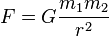# Circular Motion and Universal Law of Gravitation Solutions Check Requested!

rain_ex

## Homework Statement

1. A typical white dwarf star, which once was an average star like our Sun but now in the last stage of its evolution, is the size of our Moon but has the mass of our Sun. What is the surface acceleration due to gravity on this star? (Msun = 1.99 x 10^30 kg, Rmoon = 1.74 x 10^6 m)

- R = radius and M = mass (in reference to Msun and Rmoon)

## Homework Equations

Universal Law of Gravitation## The Attempt at a Solution

I don't know how to start, but still gave it a try.
First of all, I didn't know what the "F" on the other side of the equation is supposed to be or set the equation up to find the acceleration.
I started by substituting G for 6.67426x10^-11 and got rid of the 2nd mass altogether.
I plugged in the mass of the sun, 1.99x10^30 kg divided by the radius of the moon, 1.74x10^6 m.
Thus I ended up with 4.38x10^7 m/s^2.

Is this the solution?

We have $$F = m_2 a = G \frac{m_1 m_2}{r^2},$$
so $$a = G \frac{m_1}{r^2}.$$